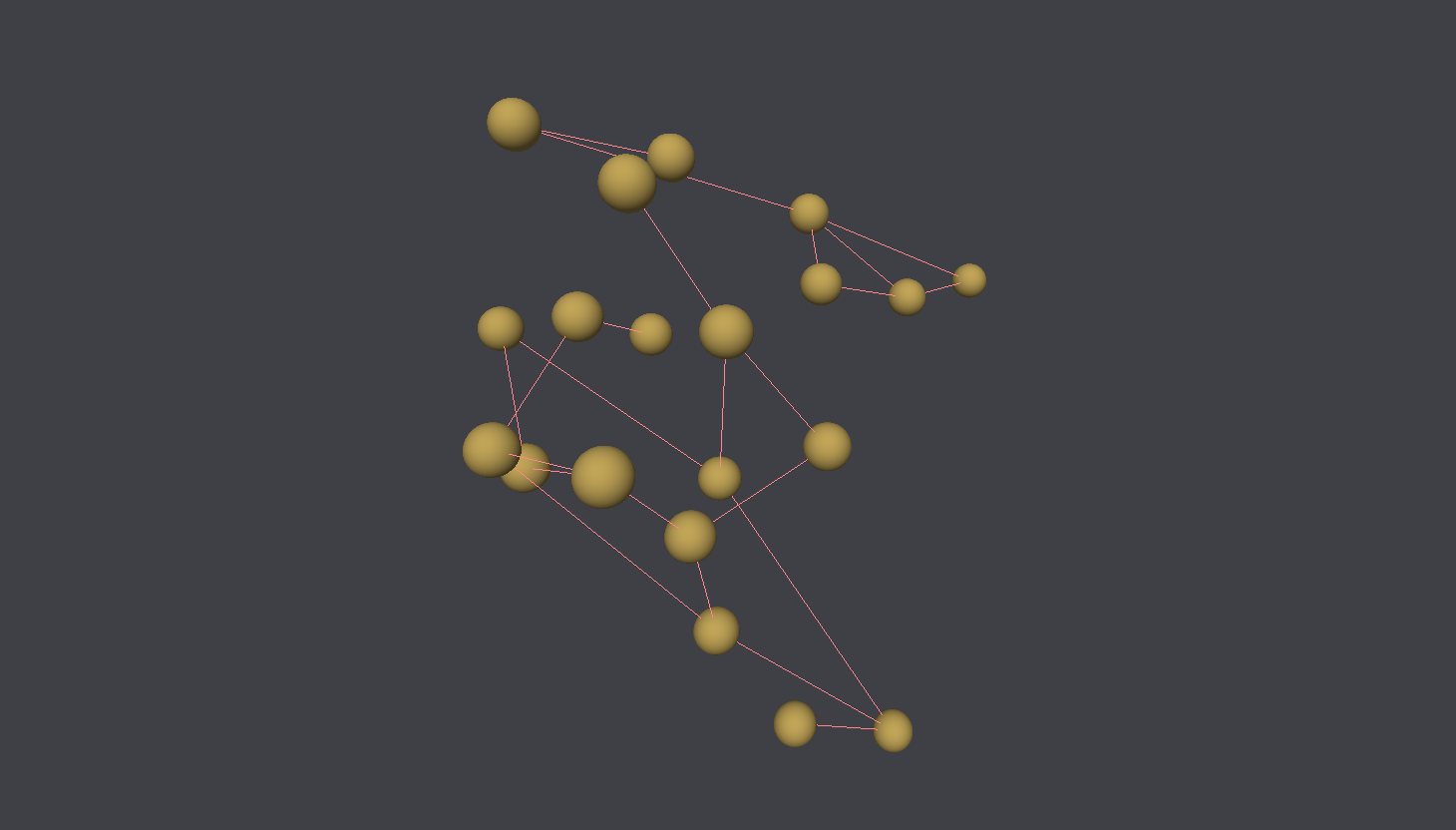Written by Øyvind Monsen

# 3D Force directed graph

As a small learning-project I made a 3d-force-directed-graph renderer that lets you explore any graph in 3D. My main motivation for this was to learn three.js.

## Force directed graph

Force-directed-graph-algorithms are a set of algorithms that tries to display graphs in an esthetically pleasing way by using concepts from physics. By applying forces between the nodes we can get connected nodes to group together and spread non-connected nodes appart, giving us a clear overview of the graph structure. There are many different such algorithms, with different results and runtimes. This first iteration I went with the clasical and most easy to uderstand approach which includes a spring-like force from edges, and electromagnetic-like repulsion.

The attraction part is as mentioned modeled after springs, which means that the force that each node feels from each edge is given by F=-kx where x is the distance between the nodes, and k is the spring-constant. If we want the springs to have an ideal length we can introduce it into the formula as follows: F=-kx/l. While this approach seems straight forward I got better results when using F=-k log(x/l) for some reason.

For repulsion the forces are similar to the repulsion of two electricly charged particles with same charge. Therefore each node feels a force from all other nodes given by F = k/x², where x is the distance between the nodes.

For all nodes we calculate the force impact from all other nodes, and then apply the force. This is done each animation frame to calculate a small change.

The resulting calcuation might give results like this:

## Optimization

These calculations can become large, especially for larger graphs. One way to optimize the runtime of each force calculation pass is to limit the nodes that we calculate the force for. The repulsion force decreases with the square of the distance, so distant nodes won't really impact each other that much. Therefore we can limit the calculation only to nodes that are within a certain distance. We do, however, not want to apply this limitation for the spring force, since we want connected clusters to collect, even though their starting point might be far appart.

Sometimes the entire graph might get a net sum force in one direction making it drift out of view, or some nodes might get to far away from the rest. To combat this we can introduce a new force: gravity. This is quite simple, each node has some attraction to the center of the scene, proportional to the distance to the center. Since the center is at (0, 0, 0) we can just apply the force as the inverse of the position vector for each node.

Lastly we also want to limit the force calculation whenever the graph comes close to some equilibrium. The equilibrium comes when the repulsion and attraction match eachother, but we can speed things up by doing aggressive calculations initially, and lower the temperature with time. To do this we multiply each force vector by some constant over the log of time.

## The code

Under you see the complete code used to calculate the force for nodes at each timestep. Then it is just a matter of calling this function each animation loop to update all the node-positions. You can also see the complete code for all the rendering over on Github.

``````1import { Vector3 } from "three";
2
3// Force per distance
4const REPULSION_FORCE: number = -0.2;
5const EDGE_FORCE: number = 0.4;
6const SIGMA: number = 0.08;
7const GRAVITY: number = 0.0002;
8let forceVectors: Vector3[] = [];
9
10export default function calculateForces(
11  nodes: THREE.Mesh[],
12  edges: number[][],
13  t: number
14) {
15  forceVectors = [];
16
17  for (let i = 0; i < nodes.length; i++) {
18    let forceVector = new Vector3();
19
20    for (let j = 0; j < nodes.length; j++) {
21      if (i === j) {
22        continue;
23      }
24      let node = nodes[i];
25      let otherNode = nodes[j];
26
27      let otherNodePosition = new Vector3(
28        otherNode.position.x,
29        otherNode.position.y,
30        otherNode.position.z
31      );
32
33      let distanceVector = new Vector3()
34        .copy(otherNodePosition)
35        .sub(node.position);
36
37      if (edges[i][j] === 1) {
38        // Attraction
39        let attraction = EDGE_FORCE * Math.log(distanceVector.length() / 0.5);
40
41        let attractionVector = new Vector3()
42          .copy(distanceVector)
43          .multiplyScalar(attraction);
44
46      } else {
47        // repulsion
48        if (distanceVector.length() < 1) {
49          let repulsion = REPULSION_FORCE / distanceVector.length() ** 2;
50          let repulsionVector = new Vector3()
51            .copy(distanceVector)
52            .multiplyScalar(repulsion);
53
55        }
56      }
57
58      // gravitation
59      let gravityVector = new Vector3()
60        .copy(node.position)
61        .multiplyScalar(-GRAVITY);